• R-squared or Coefficient of Determination
• Proportion of the variance in the dependent variable that is predictable from the independent variable(s).
• Wiki: Coefficient of determination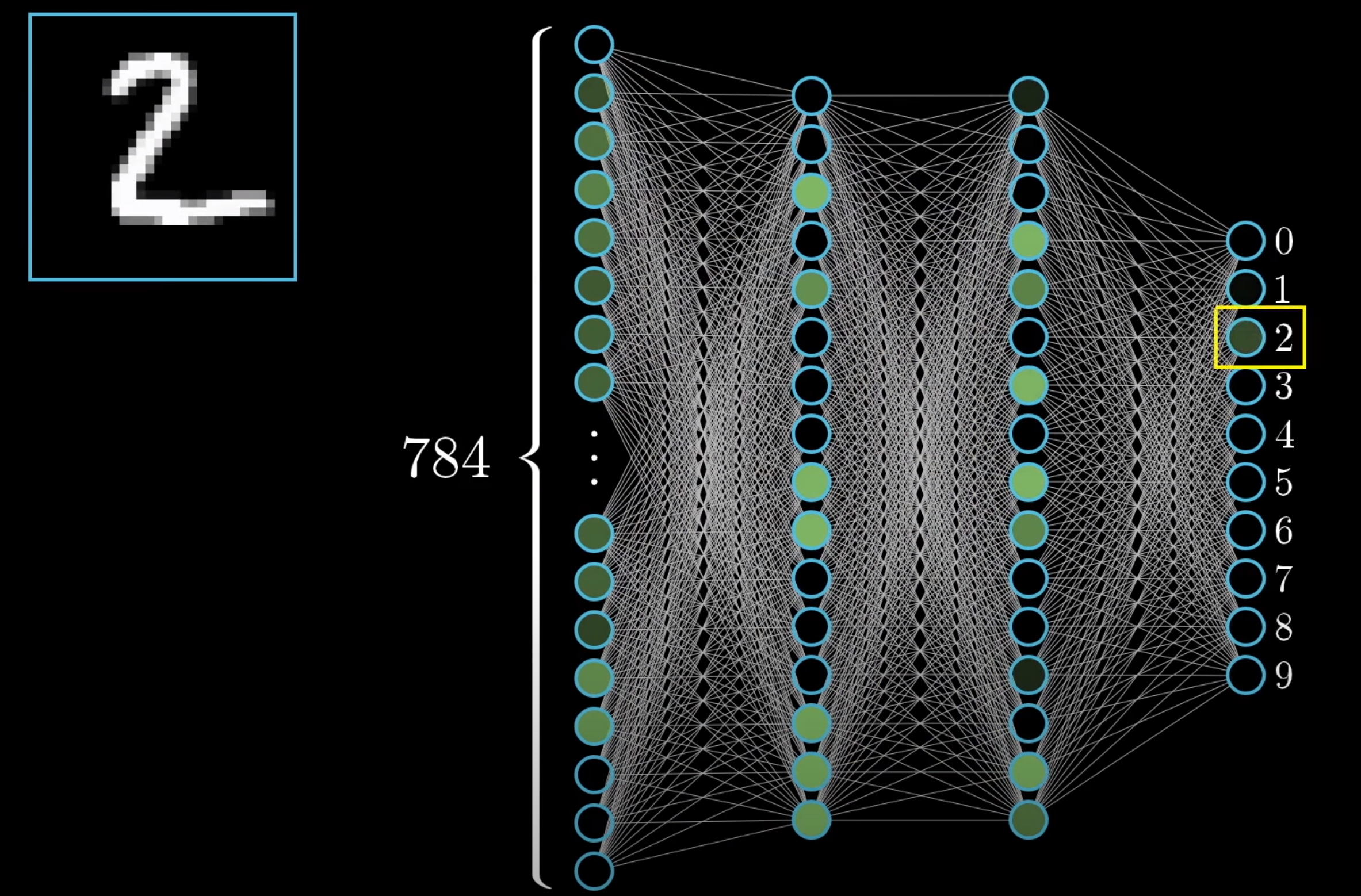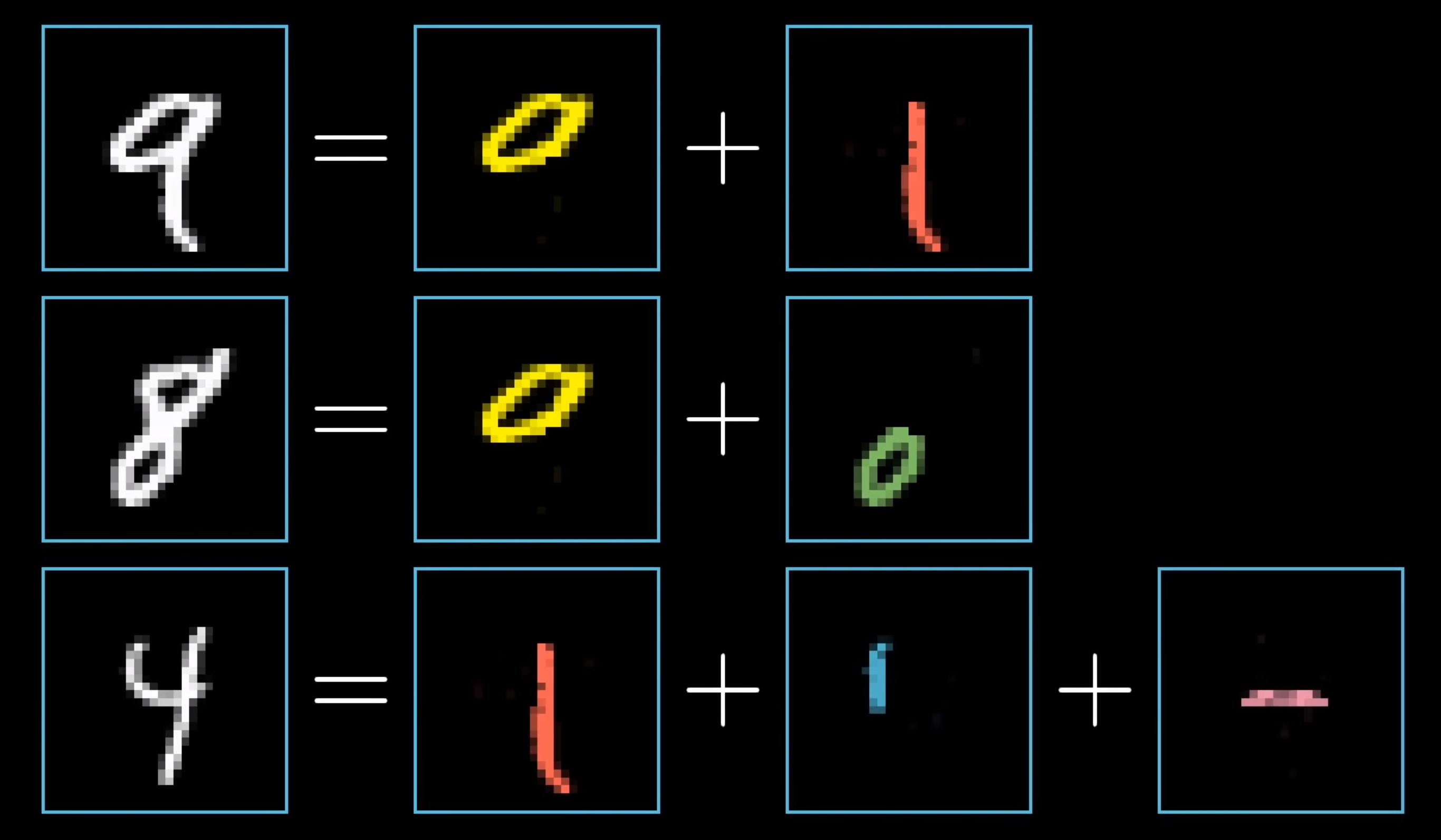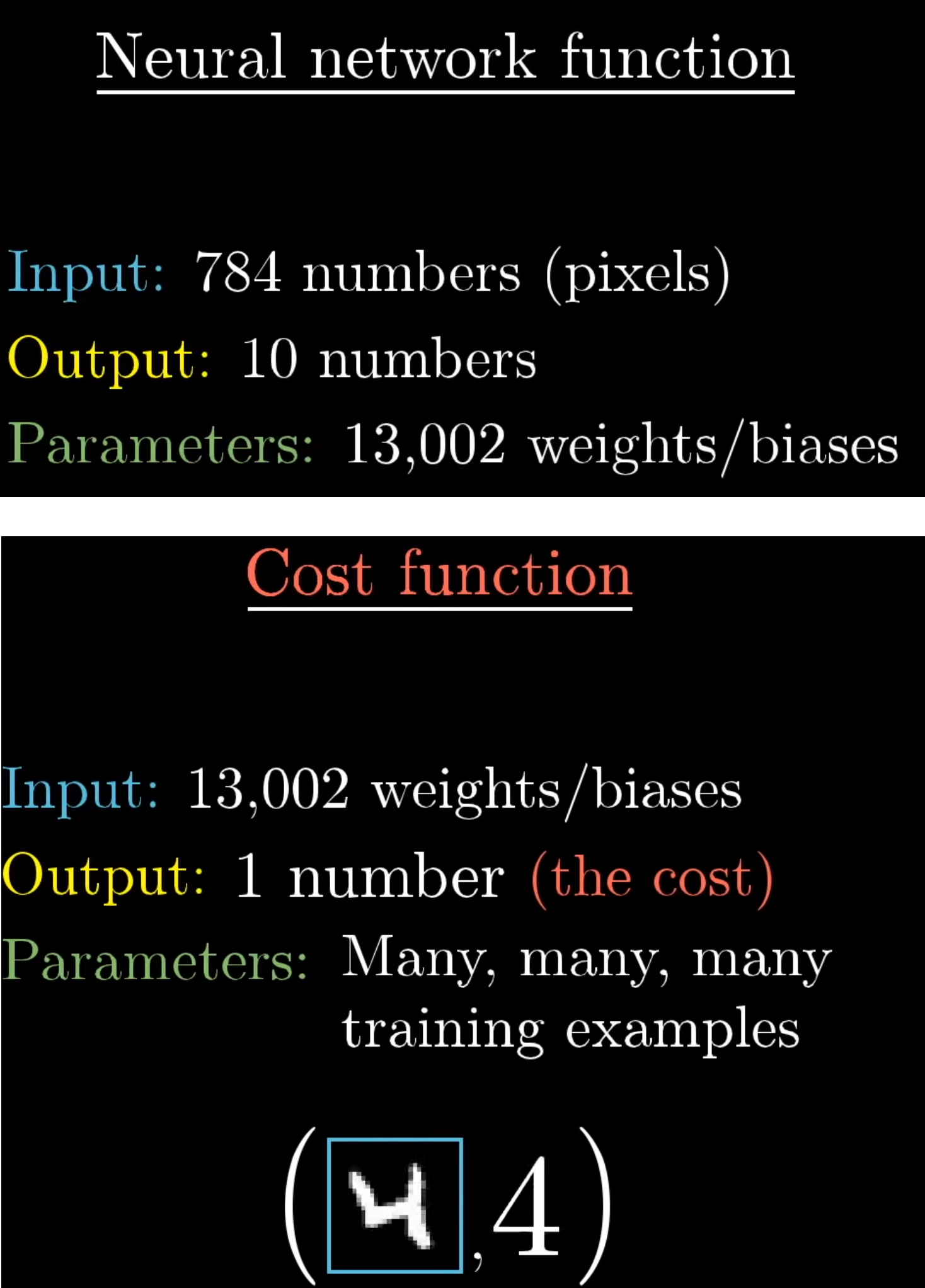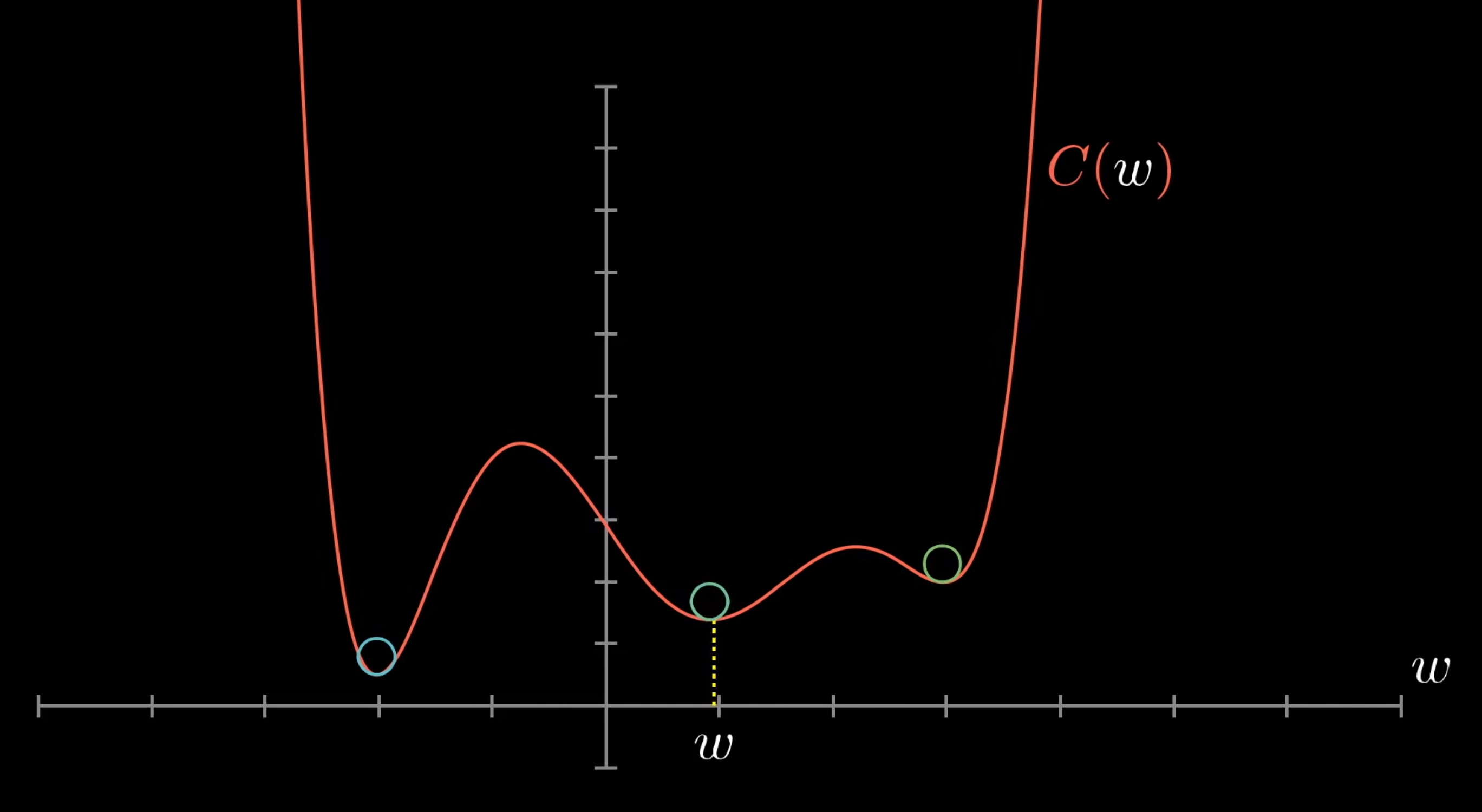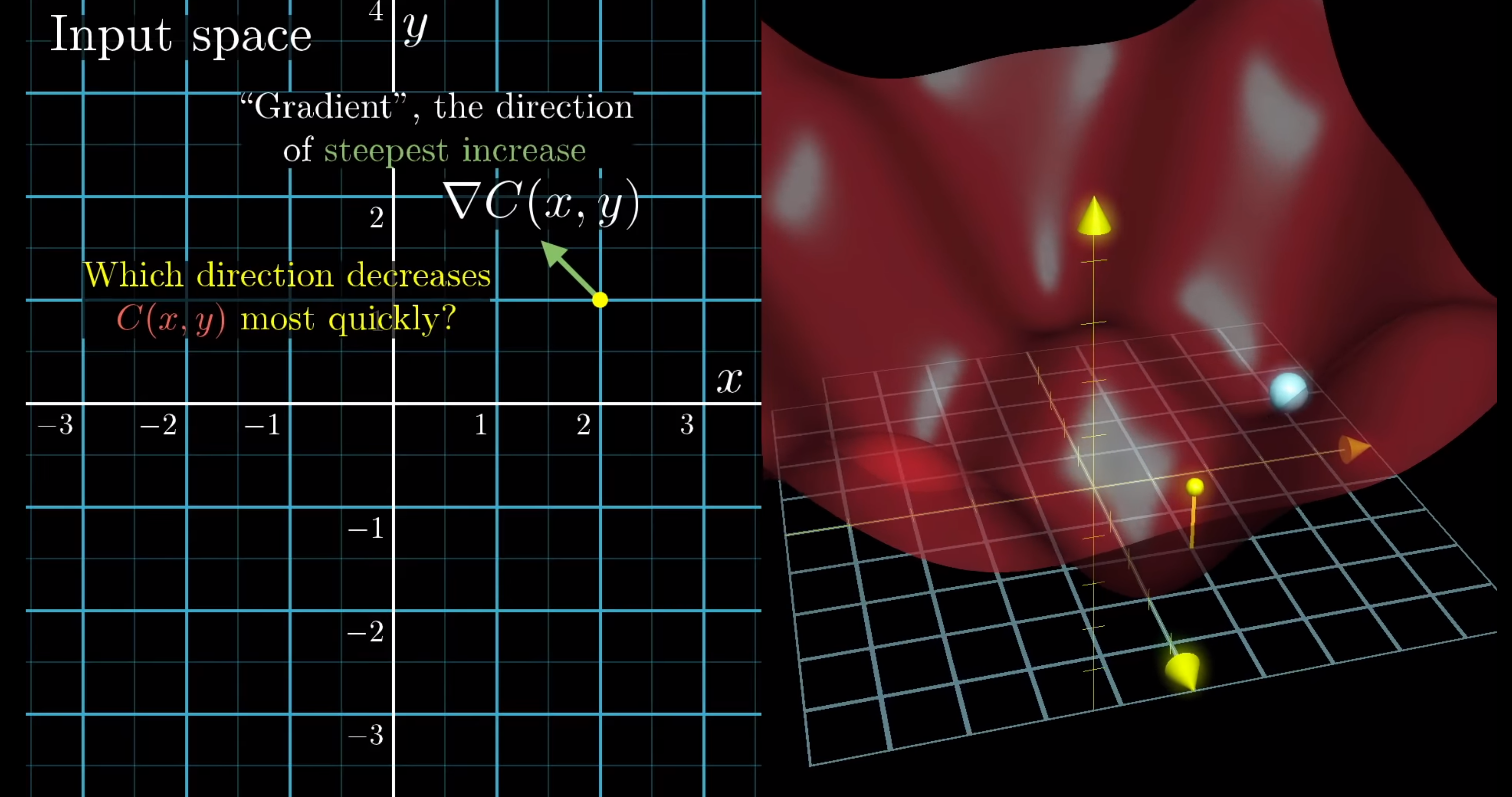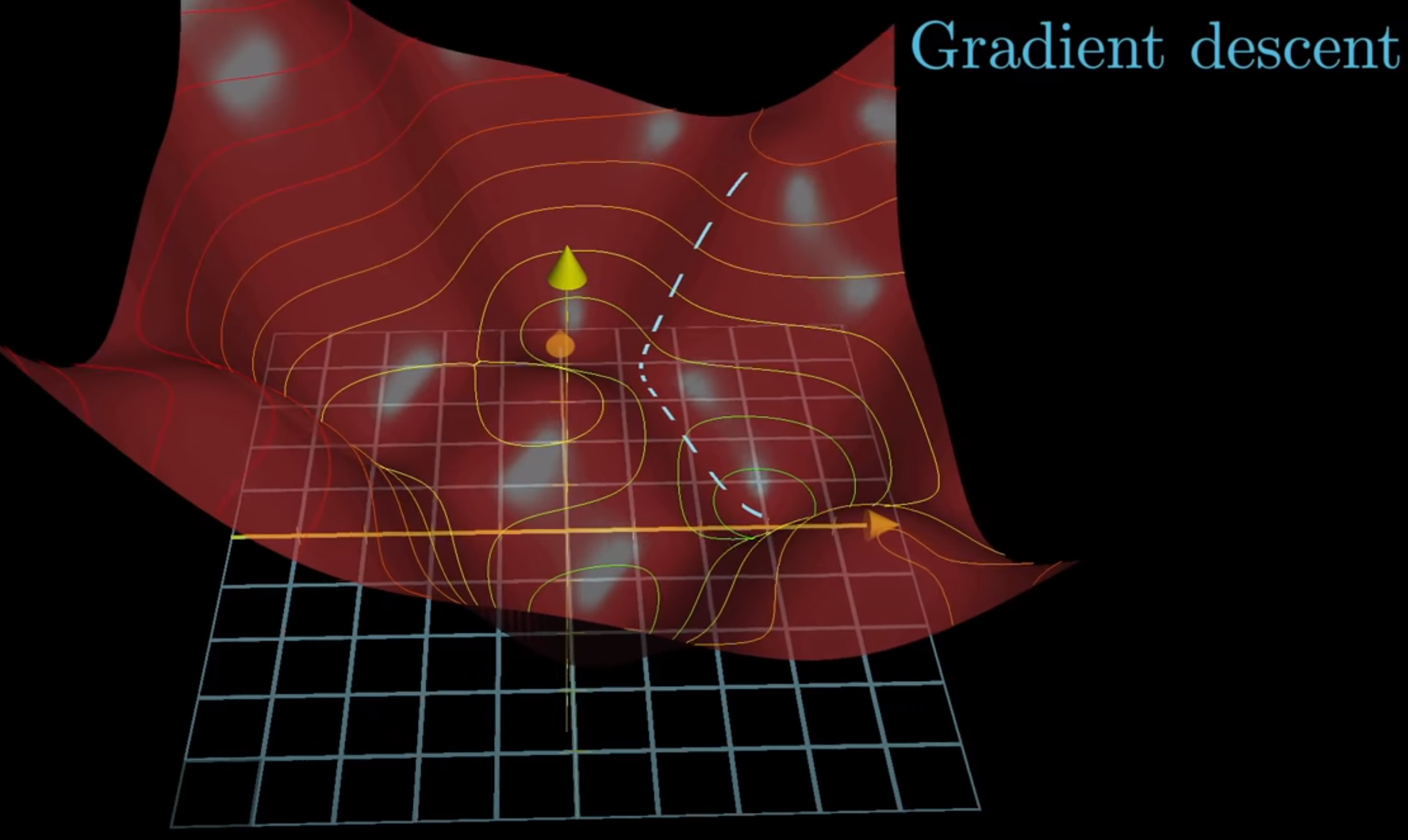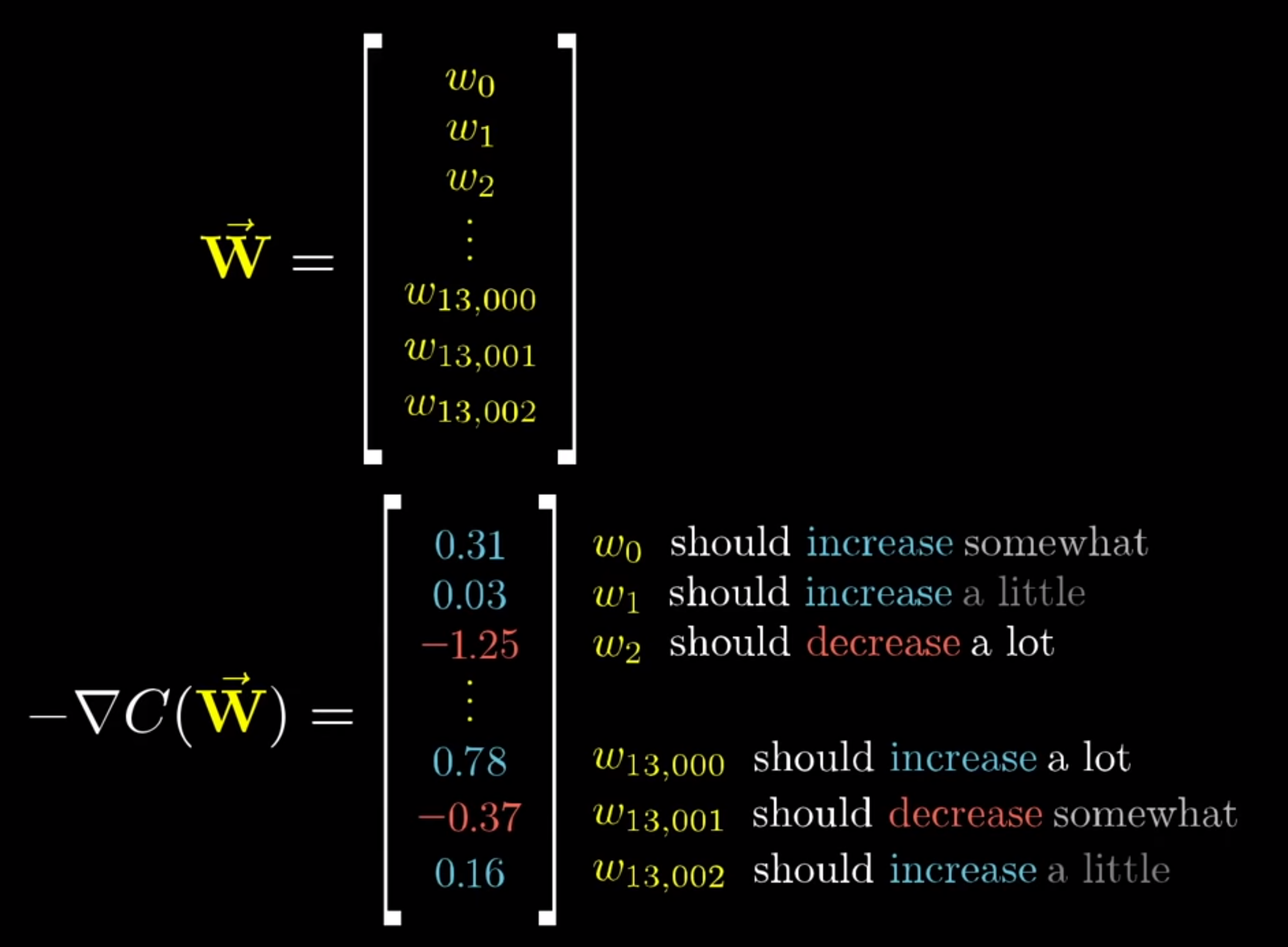• What is a `Neural Network`? Deep Learning Chapter 1 (from `3Blue1Brown`)
• Gradient descent, how neural networks learn. Deep Learning Chapter 2 (from `3Blue1Brown`)

Machine Learning - Part 6

• RoC (Receiver operating characteristic) Curve